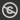Science, Maths & Technology
Free course

# Introduction to the calculus of variations## Course reviews

This free course concerns the calculus of variations. Section 1 introduces some key ingredients by solving a seemingly simple problem – finding the shortest distance between two points in a plane. The section also introduces the notions of a functional and of a stationary path. Section 2 describes basic problems that can be formulated in terms of functionals. Section 3 looks at partial and total derivatives. Section 4 contains a derivation of the Euler-Lagrange equation. In Section 5 the Euler-Lagrange equation is used to solve some of the earlier problems, as well as one arising from a new topic, Fermat’s principle.

## Course learning outcomes

After studying this course, you should be able to:

• understand what functionals are, and have some appreciation of their applications
• apply the formula that determines stationary paths of a functional to deduce the differential equations for stationary paths in simple cases
• use the Euler-Lagrange equation or its first integral to find differential equations for stationary paths
• solve differential equations for stationary paths, subject to boundary conditions, in straightforward cases.

First Published: 12/03/2018

Updated: 22/05/2018

Skip Rate and Review